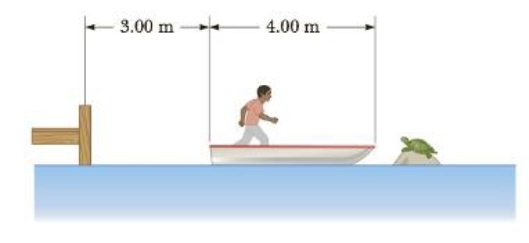Chapter 8, Problem 77AP

Chapter
Section
Textbook Problem

A 40.0-kg child stands at one end of a 70.0-kg boat that is 4.00 m long (Fig. P8.77). The boat is initially 8.00 m from the pier. The child notices a turtle on a rock beyond the far end of the boat and proceeds to walk lo that end to catch the turtle, (a) Neglecting friction between the boat and water, describe the motion of the system (child plus boat), (b) Where will the child be relative to the pier when he reaches the far end of the boat? (c) Will he catch the turtle? (Assume that he can reach out 1.00 m from the end of the boat.)Figure P8.77

(a)

To determine
The motion of the system (child plus boat) by neglecting the friction between the boat and water.

Explanation

The center of gravity of the child plus boat system remains constant hence there is no horizontal forces act on the system

The center of gravity of the system is defined as xcg=(mcxc+mbxb)/(mc+mb)=constant

(b)

To determine
The child distance relative to the pier when he reaches the far end of the boat.

(c)

To determine
It is about the catch of the turtle.

Still sussing out bartleby?

Check out a sample textbook solution.

See a sample solution

The Solution to Your Study Problems

Bartleby provides explanations to thousands of textbook problems written by our experts, many with advanced degrees!

Get Started

What do the various chromatographic separation techniques have in common?

General Chemistry - Standalone book (MindTap Course List)

Behavior change is a process that takes place in stages. T F

Nutrition: Concepts and Controversies - Standalone book (MindTap Course List)

22-7 What is the function of an immunoglobulin?

Introduction to General, Organic and Biochemistry